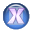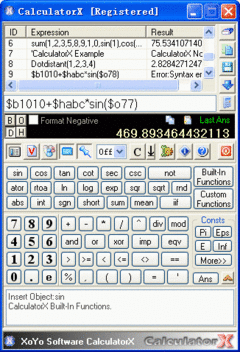Search
Software
Options for CalculatorX
CalculatorXCalculatorX is an enhanced expression calculator. It can evaluate an expression for one time. You can use Arithmetic, Logic, Bitwise and Relation operations in your expression. And many system constants and built-in functions can also be used in the expression. CalculatorX even supports the custom variables, custom functions and note-line. You can use Binary, Octal, Decimal and HEXadecimal numbers in one expression to evaluate as well.

CalculatorX has the unlimited ability to extend itself by using Custom Functions. You can add your own functions to CalculatorX in order to convenience your work.

CalculatorX is powerful, but it is very easy to use. You can use it as any calculators you have used.

Make Work Easy and High Efficiency by using CalculatorX.

Here is just a short list of CalculatorX's major features:
- Arithmetic operation
- Logic and Bitwise operation
- Relation operation
- Built-In Functions
- Constant support, such as PI, E, TRUE, FALSE etc.
- Alias for operators and functions, eg."sh" and "hsin" is the same function
- Unlimited nesting for expression
- The mixed operation for Binary, Octal, Decimal and HEXadecimal numbers
- Table/Array operation support
- "Simulate the exact value" operation
- Scientific notation for numerical value
- Custom variable support
- Note-line support
- Custom function support
- Upper case and lower case can be mixedly used in the expression
- Historical expressions log
- XP appearance support (in WinXP)
- Quick reference for input
- Base-N(2,8,10,16) results show
- Expressions manager support
- 'Auto Round Operation' according to the appointed bits